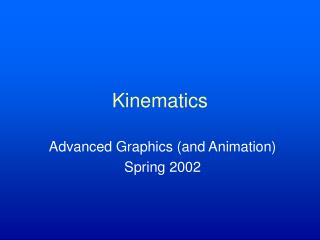DownloadDownload PresentationKinematics

# Kinematics

Télécharger la présentation## Kinematics

- - - - - - - - - - - - - - - - - - - - - - - - - - - E N D - - - - - - - - - - - - - - - - - - - - - - - - - - -
##### Presentation Transcript

1. Kinematics Advanced Graphics (and Animation) Spring 2002

2. Kinematics • The study of object movements irrespective of their speed or style of movement

3. Degrees of Freedom(DOFs) • The variables that affect an object’s orientation • How many degrees of freedom when flying? • So the kinematics of this airplane permit movement anywhere in three dimensions • Six • x, y, and z positions • roll, pitch, and yaw

4. Degrees of Freedom • How about this robot arm? • Six again • 2-base, 1-shoulder, 1-elbow, 2-wrist

5. Configuration Space • The set of all possible positions (defined by kinematics) an object can attain

6. Work Space vs. Configuration Space • Work space • The space in which the object exists • Dimensionality • R3 for most things, R2 for planar arms • Configuration space • The space that defines the possible object configurations • Degrees of Freedom • The number of parameters that necessary and sufficient to define position in configuration

7. More examples • A point on a plane • A point in space • A point moving on a line in space

8. Controlled DOFs • DOFs that you can actually control (position explicitly)

9. Hierarchical Kinematic Modeling • A family of parent-child spatial relationships are functionally defined • Moon/Earth/Sun movements • Articulations of a humanoid • Limb connectivity is built into model (joints) and animation is easier

10. Robot Parts/Terms • Links • End effector • Frame • Revolute Joint • Prismatic Joint

11. More Complex Joints • 3 DOF joints • Gimbal • Spherical (doesn’t possess singularity) • 2 DOF joints • Universal

12. Hierarchy Representation • Model bodies (links) as nodes of a tree • All body frames are local (relative to parent) • Transformations affecting root affect all children • Transformations affecting any node affect all its children

13. Forward vs. Inverse Kinematics • Forward Kinematics • Compute configuration (pose) given individual DOF values • Inverse Kinematics • Compute individual DOF values that result in specified end effector position

14. Forward Kinematics • Traverse kinematic tree and propagate transformations downward • Use stack • Compose parent transformation with child’s • Pop stack when leaf is reached • High DOF models are tedious to control this way

15. Denavit-Hartenberg (DH) Notation • A kinematic representation (convention) inherited from robotics • Z-axis is aligned with joint • X-axis is aligned withoutgoing limb • Y-axis is orthogonal

16. DH Notation • Joints are numbered to represent hierarchy • Ui-1 is parent of Ui • Parameter ai-1 is outgoinglimb length of joint Ui-1 • Joint angle, qi-1, isrotation of i-1 x-axis,xi-1, about zi-1, relativeto i-2th frame’s x-axis direction,xi-2

17. DH Notation • If nonplanar • X-axis of i-1th joint is line perpendicular to z-axes of i-1 and i frames • Link twist, ai-1, is the rotation of ith z-axis about xi-1-axis relative to z-axis of i-1th frame

18. DH Notation • Link offset, di-1, specifies the distance along the zi-1-axis (rotated by ai-1) of the ith frame from the i-1th x-axis to the ith x-axis

19. DH Notation • Not all ith variables relate to i and i-1 • Link offset (di):Distance from xi-1 to xi along zi • Joint angle (qi): angle between xi-1 and xi about zi • Link length (ai): distance from zi to zi+1 along xi • Link Twist (ai): angle between zi and zi+1 about xi

20. Screw Transformations • No, I’m not mad at them • Relationship between i+1 frame and i frame are a combination • ith joint parameters • i+1 joint parameters • Call this relationship screw transformations • Two (translation, rotation) pairs each relative to specific axis of ith and i+1 frames

21. Screw Transformations • Offset (di+1) and angle (qi+1) are translation and rotation of i+1 joint relative to ith joint w.r.t. zi-axis • Length (ai) and twist (ai) are translation and rotation w.r.t. xi-axis

22. x2 y2 O2 y0 a2 y1 2 x1 a1 O1 1 x0 O0 Planar Example

23. Ball and Socket • Model as 3 revolute joints with zero-length links between them • If all angles are set to 0, we are in gimbal lock situation (z-axes of two joints are colinear) • Instead, initialize middle joint angle to 90 degrees • … or represent using quaternions

24. Inverse Kinematics (IK) • Given end effector position, compute required joint angles • In simple case, analytic solution exists • Use trig, geometry, and algebra to solve

25. Analytic solution of 2-link inverse kinematics • Two solutions: elbow up & elbow down x2 y2 (x,y) O2 2 y0 y1 a2 2 x1  a1 O1  1 x0 O0

26. Iterative IK Solutions • Frequently analytic solution is infeasible • Use Jacobian • Derivative of function output relative to each of its inputs • If y is function of three inputs and one output • Represent Jacobian J(X) as a 1x3 matrix of partial derivatives

27. Jacobian • In another situation, end effector has 6 DOFs and robotic arm has 6 DOFs • f(x1, …, x6) = (x, y, z, r, p, y) • Therefore J(X) = 6x6 matrix

28. Jacobian • Relates velocities in parameter space to velocities of outputs • If we know Ycurrent and Ydesired, then we subtract to compute Ydot • Invert Jacobian and solve for Xdot